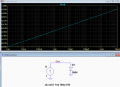# Small Signal Diodes and forward voltage

#### maanothing

Joined Sep 27, 2019
1
yet again I can find no useful answer on the popular search engine. I'm tired of the same 3 results poping up hundreds of times so out of desperation have decided to post here....

small signal diodes...mainly the 1n34a and 1ss106....do they conduct below their stated forward voltages?

what is the smallest voltage they will conduct?

James

#### MrChips

Joined Oct 2, 2009
19,280
Welcome to AAC, James!

A tricky question deserves a tricky answer.

A diode is fabricated using semi-conductors. That means that it lies somewhere between a conductor and an insulator.

A diode will always conduct current at any voltage.
How much current does a diode conduct? The diode current is given by the diode equation, also known as the Shockley diode equation. This will relate the diode current versus diode voltage at a given temperature.

So, the simple answer is, there is no minimum voltage.

#### OBW0549

Joined Mar 2, 2015
2,987
small signal diodes...mainly the 1n34a and 1ss106....do they conduct below their stated forward voltages?
Yes.

what is the smallest voltage they will conduct?
Anything above zero.

In forward-biased PN junctions, whether diode or LED or the B-E junction of BJTs, forward voltage is a logarithmic function of current-- or, to turn that around, the current is an exponential function of forward voltage.

#### crutschow

Joined Mar 14, 2008
23,366
do they conduct below their stated forward voltages?

what is the smallest voltage they will conduct?
The stated forward voltage is at a given current, but a diode has a logarithmic relation between forward voltage and current, so it will conduct down to near zero volts, just not much current.

Below is the LTspice simulation of an 1N34 germanium diode, showing this relation.
Of course the model is somewhat idealized, so an actual diode will likely look somewhat different, especially at very low currents where leakage effects can dominate.Last edited: